Home | | Maths 11th std | Combinations

# Combinations

Let us suppose there are four persons A, B, C and D (actual names may be used here) and we have to select three of them to be a part of a committee.

Combinations

Let us suppose there are four persons A, B, C and D (actual names may be used here) and we have to select three of them to be a part of a committee. In how many ways can we make this selection? For example, A, B, C is one possible choice. Here the order of selection is immaterial. Thus A, B, C is the same as B, A, C or C, A, B as long as the same three persons are selected. Thus the possible distinct choices or selections are A, B, C; A, B, D; A, C, D and B, C, D. We may thus conclude that there are 4 ways of selecting 3 people out of 4. Each choice or selection is referred to as a combination of 4 different objects taken 3 at a time.

Suppose two persons are to be selected from four persons. The possible choices are: A, B : A, C: A, D : B, C : B, D : C, D. Thus the number of combinations of 4 different objects taken 2 at a time is 6. The number of combinations of n different objects taken r at a time is represented by nCr. From the above we may conclude that 4C3 = 4 and 4C2 = 6. Now, 4C3 is the number of combinations of 4 objects taken 3 at a time. Note that in each combination, the three objects may be arranged in 3! ways.

Thus the total number of permutations of 4 objects taken 3 at a time is 4C3 × 3!. This is also equal to 4P3. Hence 4P3 =4 C3 × 3!.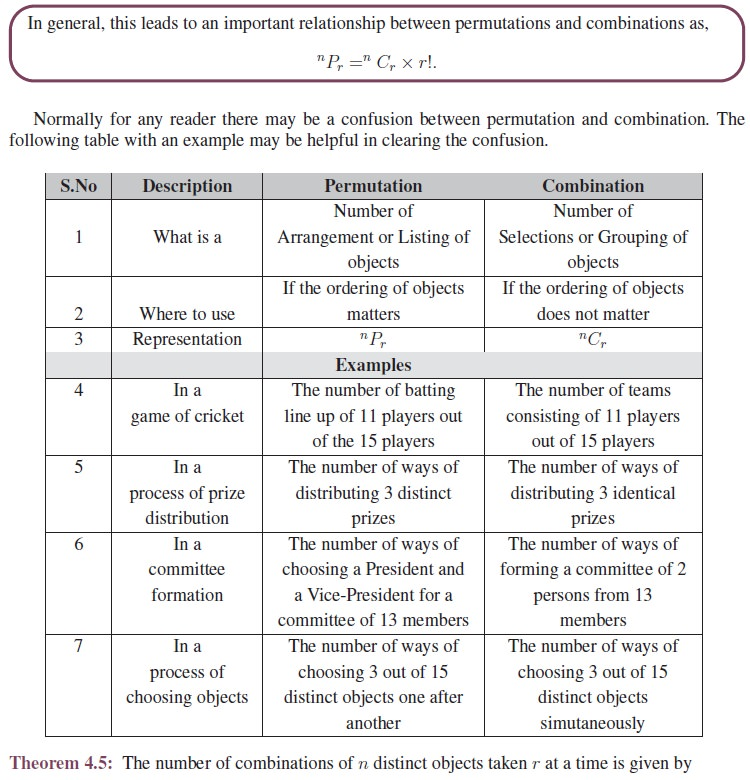## 1. Properties of Combinations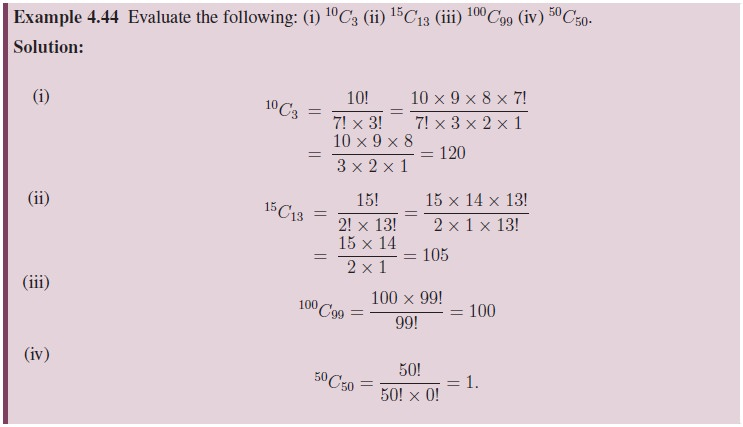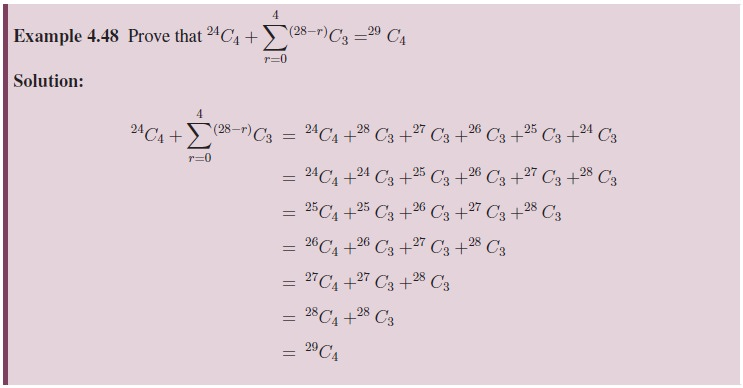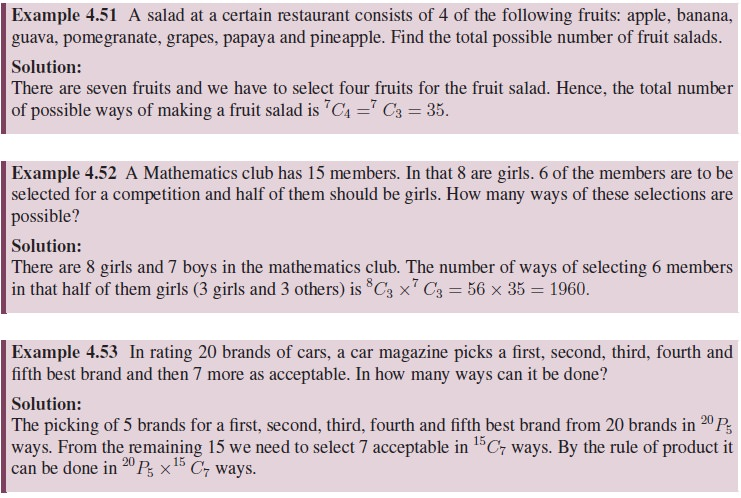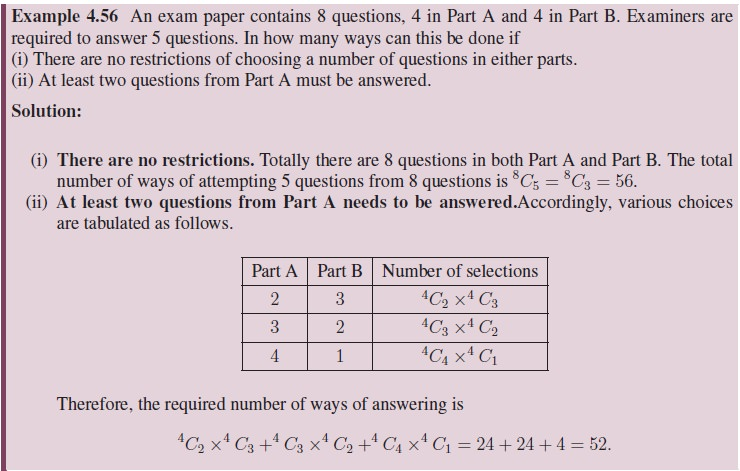Tags : Definition, Formula, Solved Example Problems, Exercise | Mathematics , 11th Mathematics : UNIT 4 : Combinatorics and Mathematical Induction
Study Material, Lecturing Notes, Assignment, Reference, Wiki description explanation, brief detail
11th Mathematics : UNIT 4 : Combinatorics and Mathematical Induction : Combinations | Definition, Formula, Solved Example Problems, Exercise | Mathematics